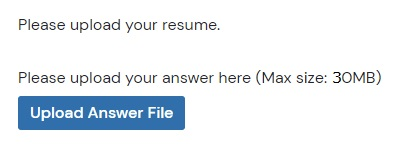## General question scoring principles

All question types follow the same scoring principles:

• Correct answers are given maximum points (100%).

• Wrong answers, or questions that auto-submitted without an answer because the time expired, are given zero points (0%).

• When possible, partial answers are given partial scores, where each correct answer gives an equal amount of points.

• Random guessing is discouraged by deducting points for wrong answers in questions where the answer can be randomly guessed.

• The total question score can't be negative, even if a candidate answered everything incorrectly.

## Total test score

The test score is the sum of scores for each question:

TestScore = ScoreQ1 + ScoreQ2 + ... + ScoreQN

Note that each question can have a different maximum score. TestDome questions have between three and ten points, but you can override each question's maximum points in your test. Simply click on the points in the Questions tab of the test, and you’ll be able to select a new value as shown in the image:For each question type, we will give an explanation, a mathematical formula, and an example.

## Multiple Choice Questions (MCQ)

MCQ questions allow selecting only one answer. The scoring formula is:

MCQScore = 100% (MaxScore) if the correct answer is selected; 0% (0) otherwise.

Example:If the candidate selects “4,” they will score maximum points (100%). If they select any other answer, their score will be zero (0%).

MCA questions have multiple correct answers, and our formula deducts points for wrong answers. Otherwise, it would be possible for candidates to select all answers and score 100% on the question. The scoring formula is:

MCAScore = selectedCorrect / totalCorrect - selectedWrong / totalWrong

Example:To score 100% on this question a candidate needs to select all three correct answers (4, 6, and 8), and not select any incorrect answers (5 and 7).

If a candidate selects two correct answers and one wrong answer, their score will be: (2/3) - (1/2) = 0.166 = 17%.

If a candidate selects two correct answers, but doesn’t select the third correct answer and doesn’t select any wrong answers, their score will be: (2/3) - (0/2) = 0.666 = 67%.

## Multiple MCQ (MMCQ)

This is a combination of MCQ and MCA type questions. There are multiple MCQ or MCA sub-questions, and each of these sub-questions is scored the same as MCA questions. The overall score is a sum of scores for each sub-question, divided by the total number of sub-questions. The scoring formula is:

MMCQScore = (SubQ1Score + SubQ2Score + … + SubQNScore) / TotalSubQuestions

Example:If a candidate selects the correct answers for each sub-question (2, 4, and 6), they will score 100%.

If a candidate instead selects the correct answers for the first 2 sub-questions, but selects the wrong answer for the third sub-question, their score will be (1/1 * 1/3) + (1/1 * 1/3) + (-1/3 * 1/3) = 0.56 = 56%

## Live Coding (CODE)

Each live coding question has several test cases whose conditions need to be satisfied to score 100%. The test cases are specific to each question. The scoring formula is:

CODEScore = CorrectTestCases / TotalTestCases

Example:If a candidate's solution passes all three test cases, they will score 100% on the question. If their solution passes one test case but fails the other two, their score will be: 1/3 = 0.33 = 33%

## File Upload with Validator (FILEV)

File Upload with Validator questions are scored the same as live coding questions — the have test cases whose conditions need to be satisfied to score 100%. The scoring formula is:

FILEVScore = CorrectTestCases / TotalTestCases

This question type requires candidates to upload an answer file, rather than typing in their answer directly. FILEV questions may also have a template file that candidates need to make changes to and then re-upload as their answer.

Example:If a candidate's answer file passes both test cases, they will score 100% on the question. If the file passes one test case but fails the other, they will score: 1/2 = 0.5 = 50%

## Number Picker (NUM)

This question type asks the candidate to enter a number into the answer box. If the answer entered in the box matches the correct answer, the score is 100%. If the answer does not match the correct answer, the score will be 0%. The scoring formula is:

NUMScore = 100% if the correct answer is entered; 0% otherwise

Example: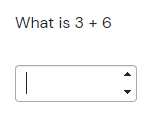If the candidate enters “9” into the answer box, they will score 100% on the question. If they enter anything other than the number “9”, their score will be 0%

## Fill The Blanks (FILL)

This type of question has two different subtypes: free form text and drop-down. Free form text requires filling a blank box with text or a number. Drop-down lets the candidate select one answer from a drop-down menu. Each blank adds to the total score, divided by the number of blanks in the question. The scoring formula is for the free form text version is:

FILLScore = CorrectBlanks / TotalBlanks

Example 1 (free form text):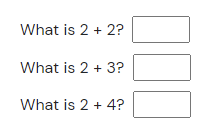A candidate will score 100% only if they fill in all answers correctly (4, 5, and 6). If they fill in the first two blanks correctly but fail the third blank, they will score: (1/3) + (1/3) + (0/3) = 0.666 = 67%

The scoring formula for the drop-down menu version is:

FILLScore = CorrectBlanks / TotalBlanks - (1 / NumberOfIncorrectOptions) / TotalBlanks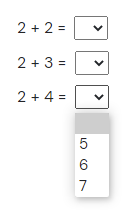If a candidate selects the correct answers for all blanks, they will score 100%. If they instead choose the correct answer for one blank but the wrong answer for the other two, they will score: (1 * 1/3) + ((-1 / 2) / 3) + ((-1 / 2) / 3) = 0 = 0%

## Quality Assurance (QA)

Quality Assurance questions have test cases that a candidate’s solution needs to pass to score 100%. The scoring formula is:

Effectiveness = BugsFound / (TotalBugs + IncorrectTestCases)

Efficiency = MinimumTestCases / SubmittedTestCases * BugsFound / TotalBugs

QAScore = Effectiveness * Efficiency

It’s important to note that:

• If SubmittedTestCases is equal to 0, Efficiency will also be 0.
• The upper limit for Efficiency is 1.

Example: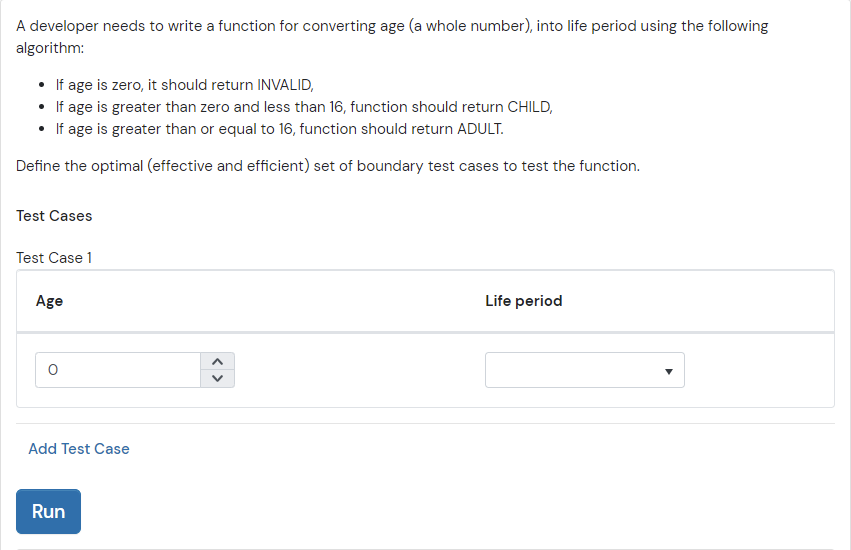Text Answer questions require candidates to type in an answer. Unlike other question types, TEXT questions are not scored automatically. You need to score them manually after a candidate completes the test.

Example: